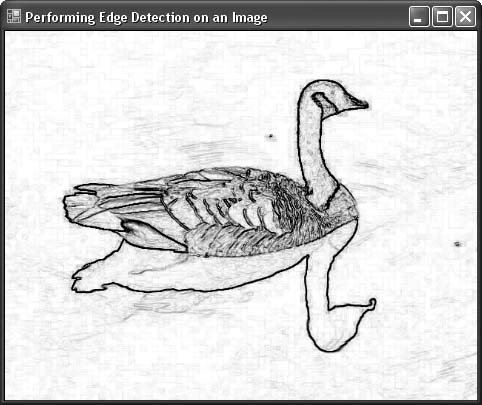# Recipe 10.20. Performing Edge Detection on an Image

#### Problem

You want to perform edge detection on a picture.

#### Solution

Sample code folder: Chapter 10\LockImage

Use the EdgeDetect() method of the LockImage class, described in Recipe 10.18.

#### Discussion

Edge detection is a good example of the complex image-processing routines that can be created within the framework of the LockImage class. The EdgeDetect() method processes the pixels in an image by converting them to grayscale and then using a filter matrix to process neighboring pixels. The matrix processing detects rapid rates of change in the pixels and assigns a darker shade of gray where pixels are changing the fastest. Figure 10-30 shows the edges of the goose after this method has done its work.

##### Figure 10-30. Edge detection using the LockImage class's EdgeDetect( ) methodThe EdgeDetect() method is a little more involved than the image-processing methods discussed in the previous two recipes. Two 3 x 3 matrices, edgeX and edgeY, are created to process neighboring pixels for X and Y changes. This processing requires that the pixels be accessed multiple times. It is easier to set up the algorithm by first converting all pixels to shades of gray and storing them in a two-dimensional array. Even with these extra processing steps, the algorithm runs very fast in the .NET Framework.

Here's the code for the EdgeDetect( ) procedure:

` Public Sub EdgeDetect( )    ' ----- Enhance the edges within the image.    Dim onePixel As Integer    Dim redPart As Integer    Dim greenPart As Integer    Dim bluePart As Integer    Dim maxColor As Integer    Dim minColor As Integer    Dim down As Integer    Dim across As Integer    Dim pixArray(,) As Integer    Dim target(,) As Integer    Dim sumX As Integer    Dim sumY As Integer    Dim useSum As Integer    Dim squareX As Integer    Dim squareY As Integer    ' ----- Define the Sobel Edge Detector gradient    '       matrices.    Dim edgeX(,) = {{-1, 0, 1}, {-2, 0, 2}, {-1, 0, 1}}    Dim edgeY(,) = {{1, 2, 1}, {0, 0, 0}, {-1, -2, -1}}    ' ----- Lock the image for speed.    LockTheImage( )    ' ----- Convert the 1D pixel array to 2D for ease    '       of processing.    ReDim pixArray(BaseImageHeight - 1, BaseImageWidth - 1)    For down = 0 To BaseImageHeight - 1       For across = 0 To BaseImageWidth - 1          ' ----- Convert each pixel to a grayscale value.          onePixel = ImageBuffer(down * BaseImageWidth + _             across)          redPart = (onePixel >> 16) And &HFF          greenPart = (onePixel >> 8) And &HFF          bluePart = onePixel And &HFF          maxColor = Math.Max(redPart, Math.Max(greenPart, _             bluePart))          minColor = Math.Min(redPart, Math.Min(greenPart, _             bluePart))          pixArray(down, across) = (maxColor + minColor) \ 2       Next across    Next down    ' ----- Results will be placed in a second pixel array.    ReDim target(BaseImageHeight - 1, BaseImageWidth - 1)    ' ----- Process for   edge detection.    For down = 0 To BaseImageHeight - 1       For across = 0 To BaseImageWidth - 1          ' ----- Calculate the edge factor.          sumX = 0          sumY = 0          If (down = 0) Or _                (down = (BaseImageHeight - 1)) Then             ' ----- Ignore true   edges.             useSum = 0          ElseIf (across = 0) Or _                (across = (BaseImageWidth - 1)) Then             ' ---- Ignore true edges.             useSum = 0          Else             ' ----- Summarize a small square around             '       the point.             For squareX = -1 To 1                For squareY = -1 To 1                   sumX += pixArray(down + squareY, _                      across + squareX) * _                      edgeX(squareX + 1, squareY + 1)                   sumY += pixArray(down + squareY, _                      across + squareX) * _                      edgeY(squareX + 1, squareY + 1)                Next squareY             Next squareX             ' ----- Force the value into the 0 to 255 range.             useSum = Math.Abs(sumX) + Math.Abs(sumY)             If (useSum < 0) Then useSum = 0             If (useSum > 255) Then useSum = 255             useSum = 255 - useSum             ' ----- Save it as a grayscale value in             '       the pixel.             target(down, across) = useSum + _                (useSum << 8) + (useSum << 16)          End If       Next across    Next down    ' ----- Move results back into the locked pixels array.    For down = 0 To BaseImageHeight - 1       For across = 0 To BaseImageWidth - 1          ImageBuffer(down * BaseImageWidth + across) = _             target(down, across)       Next across    Next down    ' ----- Finished. Unlock the image.    UnlockTheImage( ) End Sub `

Recipe 10.18 describes the LockImage class used in this recipe. Recipe 10.21 includes the full source code for the LockImage class.Visual Basic 2005 Cookbook: Solutions for VB 2005 Programmers (Cookbooks (OReilly))
ISBN: 0596101775
EAN: 2147483647
Year: 2006
Pages: 400